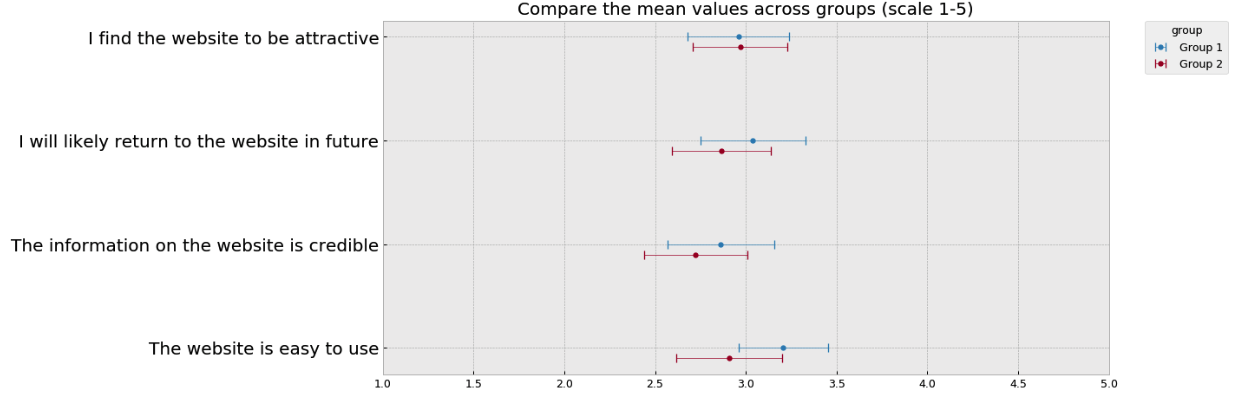## Introduction

Survey Monkey is an online survey software that helps you to create and run online surveys. It is also possible to visualize the survey results in Survey Monkey (for a single survey). However, if we want to compare the same question across several different surveys, it is difficult to do so directly in Survey Monkey. Luckily, we can solve the problem by using programming languages like Python.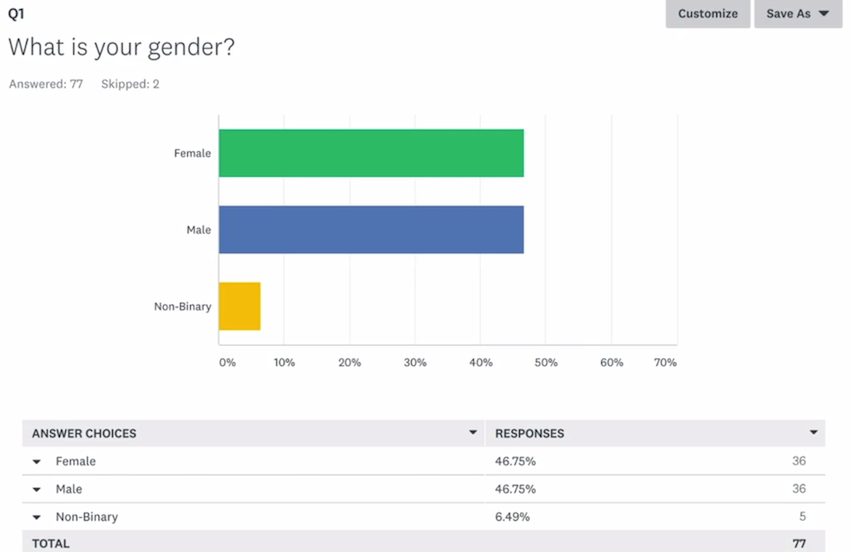The reason why I wrote this blog post is to share knowledge. I am still at the stage of learning python for data analysis. When I first encountered this problem of analyzing survey monkey data in python, I tried to google if there is anyone else who has shared a solution. I failed to find any direct answers on the first page of google. After trials and errors, I finally came up with some tricks and useful functions to solve the problem. Therefore, I want to share my knowledge and python functions which might help others to save some time.

This post will show you how to analyze survey data exported from Survey Monkey from multiple surveys in Python. To be more specific, I will first explain how to import Survey Monkey data into Python and automatically generate a codebook, and then share my code for visualizing the survey results for three types of survey questions:

• matrix table

For all the codes below, I used python 3.7 and the following packages:

#pandas==0.24.2
import pandas as pd
#numpy==1.16.2
import numpy as np
#seaborn==0.9.0
import seaborn as sns
#matplotlib==3.0.3
import matplotlib.pyplot as plt
#set plot style
plt.style.use('bmh')


You can see the complete code for all my analysis in this notebook.

## Import Survey Monkey data into Python

### Step 1: Export data from Survey Monkey

I first created an example survey on Survey Monkey including the aforementioned three types of questions. I also manually created some fake responses in order to demonstrate how the raw data looks like after exporting from Survey Monkey.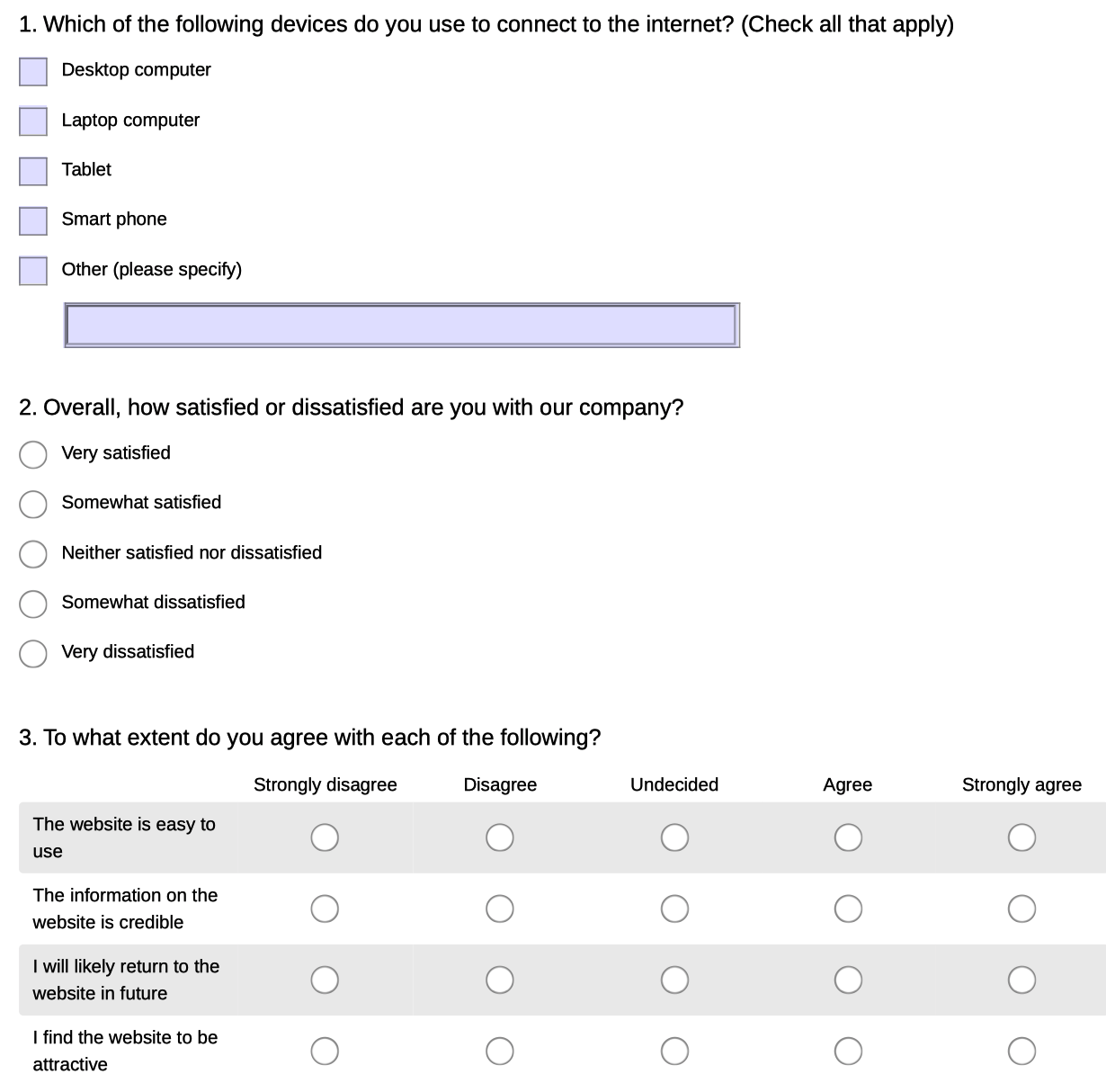You can export data from Survey Monkey easily by going to the “Analyze results” tab in a Survey and then click ”Save as”…”All response data”. It is recommended to export the “Actual answer text” so that you do not need to prepare the codebook manually.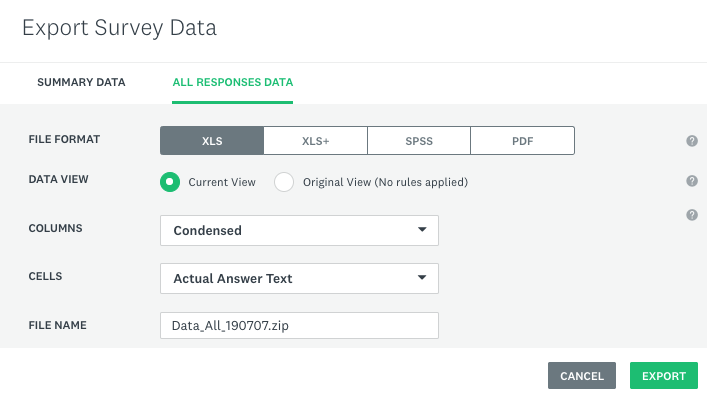Please export all the survey data that you want to merge later in Python from Survey Monkey, rename the CSV files inside the zip file, and move them into the working directory.

### Step 2: Import Survey Monkey data into Python

We can use the pandas package to import the data as a dataframe. Please note the data exported from Survey Monkey actually includes two rows of headers. They can be used as a codebook to better understand the meaning of each column.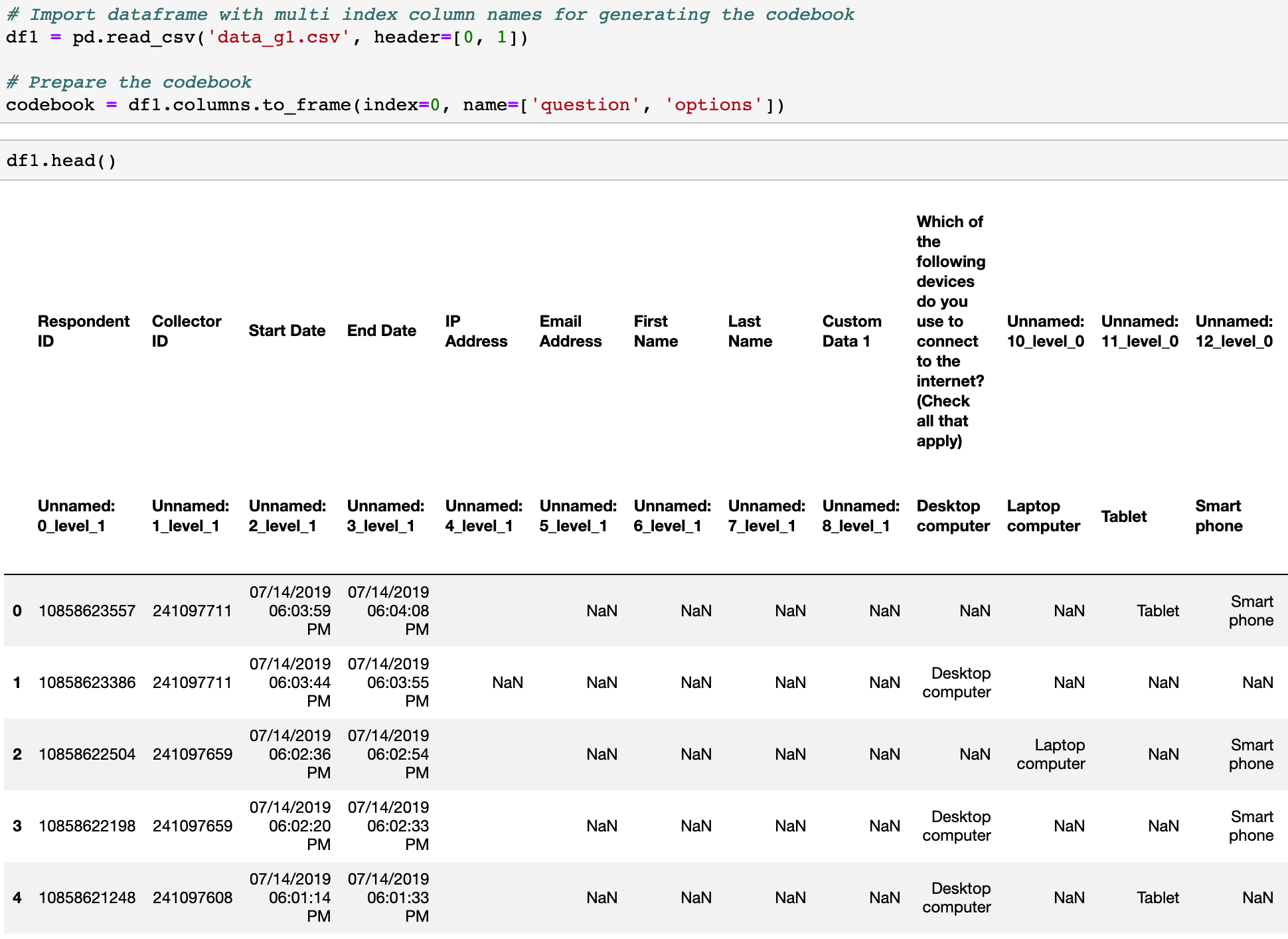As we can see from the screenshot above, the original column names are very complicated. In order to easily refer to each column later in the data analysis process, I decided to replace the header in a dataframe using numerical values with a prefix of “Q”.

In the following screenshot, you can see that I imported two datasets from two surveys that share the same question and merged them into one dataframe.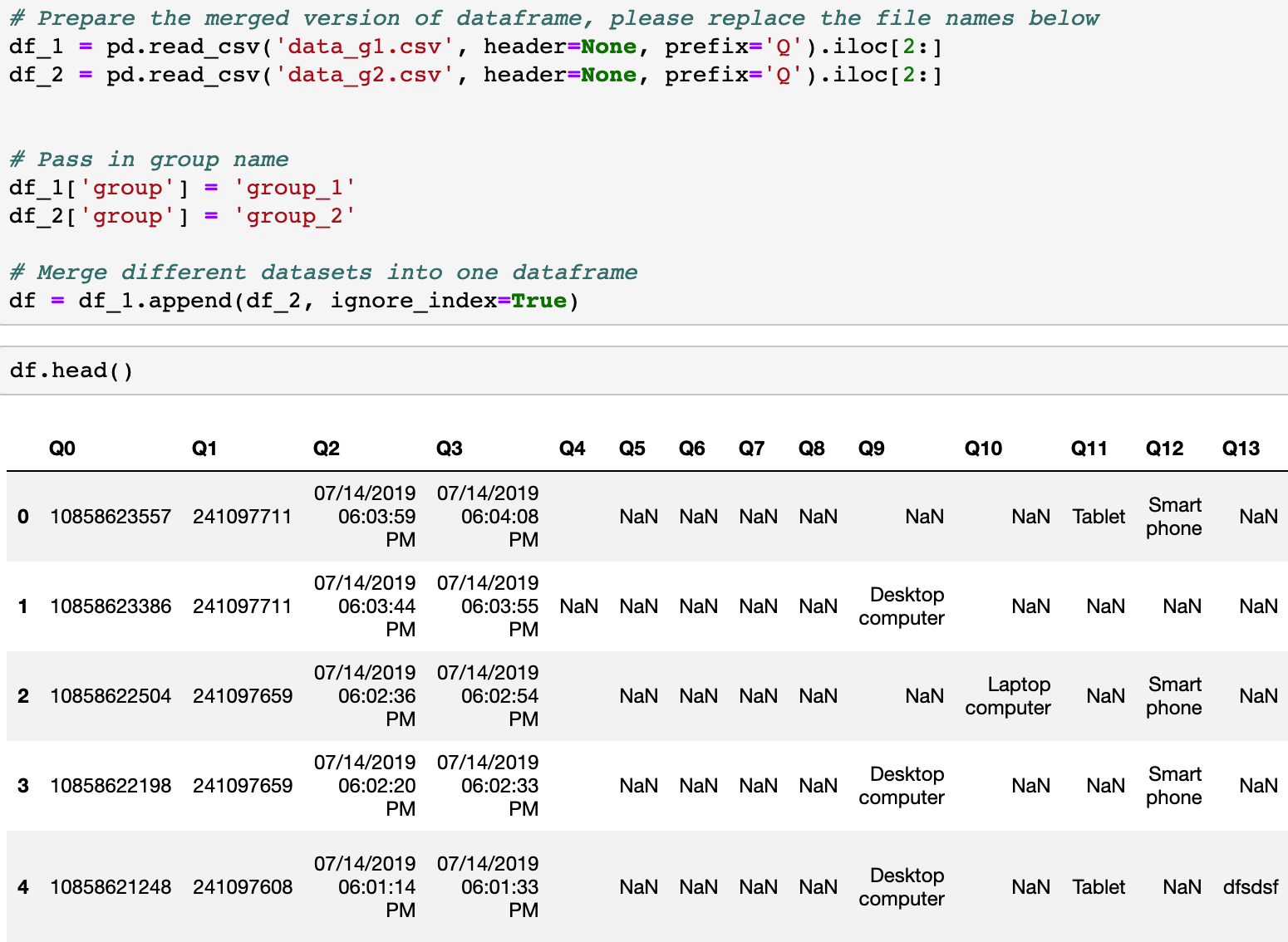## Data visualization by group

In order to demonstrate the use of the following functions, I created more fake data with the python library Faker.

### How to visualize the multi-answer question by group?

Example code:

# Prepare the summary table (please clean the data beforehand)
def prepare_table(data, column_range, group_column_name='group'):
res = []
col_range_index = list(column_range)
group_index = data.columns.to_list().index(group_column_name)
col_range_index.append(group_index)
series = data.iloc[:, col_range_index].groupby([group_column_name]).count().unstack()
for group in series.index.levels:
for var in series.index.levels:
res.append(
[
(series[var][group] / data.loc[:,group_column_name].value_counts()[group] * 100).round(2),
codebook.iloc[int(var[1:]), 1],
group,
]
)
return pd.DataFrame(columns=["percentage(%)", "options", group_column_name], data=res)

# Generate the checkbox chart based on the summary table
def gen_chart_checkbox(data, column_range, group_column_name='group'):
listOfGroup = list(data.loc[:,group_column_name].unique())
listOfGroup.sort()
table_sum = prepare_table(data, column_range, group_column_name)

print("Number of answers in each group: ")
print(data.loc[:,group_column_name].value_counts())

fig, ax = plt.subplots(figsize=(10, 8))
ax = sns.barplot(
x="percentage(%)",
y="options",
hue=group_column_name,
hue_order=listOfGroup,
data=table_sum,
)
ax.set(xlim=(0, 100))
ax.set_ylabel("")
plt.title(codebook.iloc[column_range, 0], fontsize=15)
return plt.show()



Input:

• data: Name of the dataframe
• column_range: A list of columns for the multi-answer questions
• group_column_name: The name of the column to identify group in the string format

Output: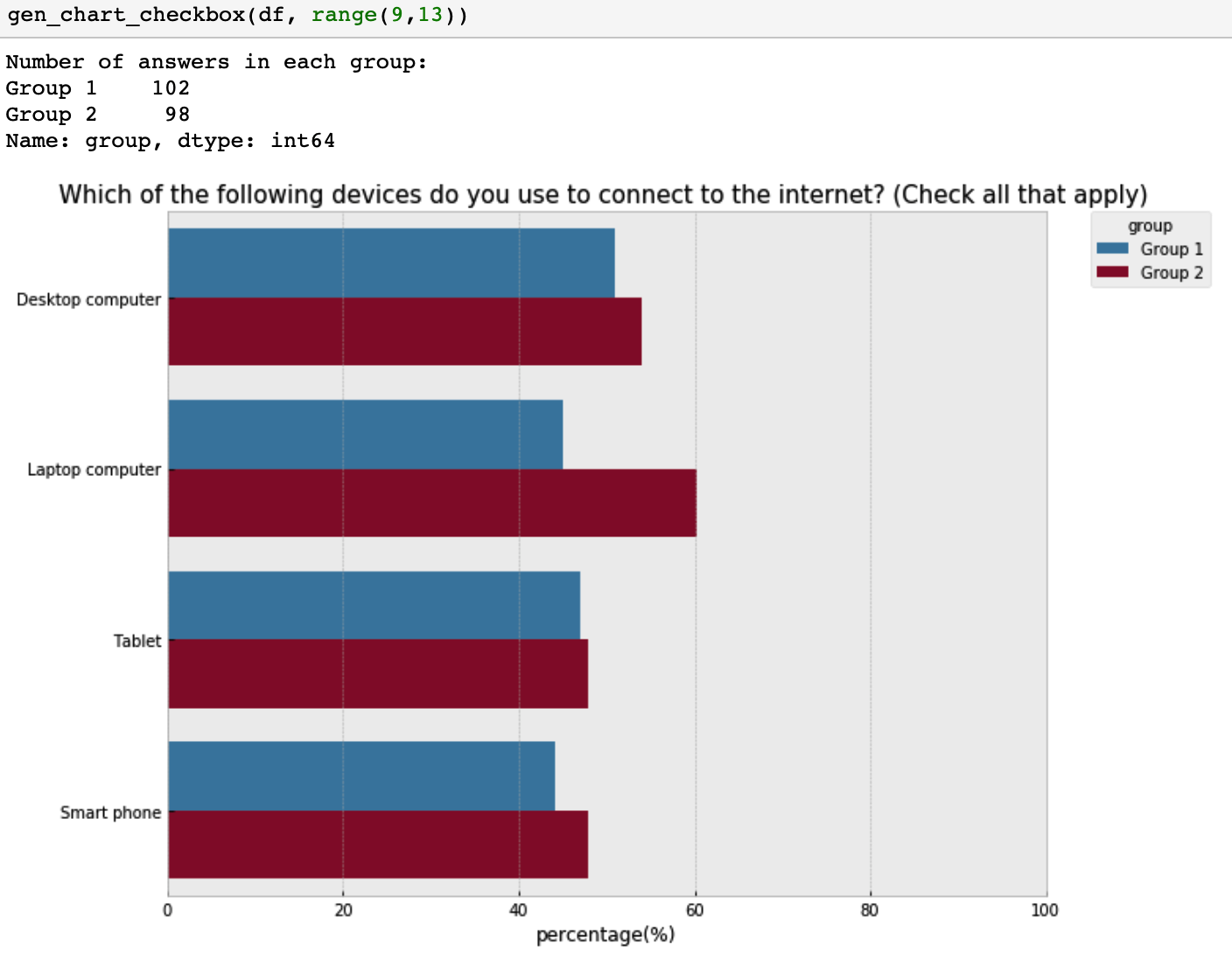### How to visualize the single-answer question by group?

Example code:

# Generate a chart to visualize single-answer questions
print("Number of answers in each group: ")
print(data[[question_name, group_column_name]].groupby(group_column_name).count())
i_counts = (
data.groupby([group_column_name])[question_name]
.value_counts(normalize=True)
.rename("percentage(%)")
.mul(100)
.reset_index()
.round(2)
)

listOfGroup = list(data.loc[:,group_column_name].unique())
listOfGroup.sort()

fig, ax = plt.subplots(figsize=(10, 8))

fig = sns.barplot(
x="percentage(%)",
y=question_name,
order=index,
hue=group_column_name,
hue_order=listOfGroup,
data=i_counts,
)

plt.title(codebook.iloc[int(question_name[1:]), 0])
ax.set(xlim=(0, 100))
ax.set_ylabel("")
return plt.show()


Input:

• data: Name of the dataframe
• question_name: Column name of the question
• index: a list of the categorical labels in an order that makes the most sense for understanding the chat
• group_column_name: The name of the column to identify group in the string format

Output: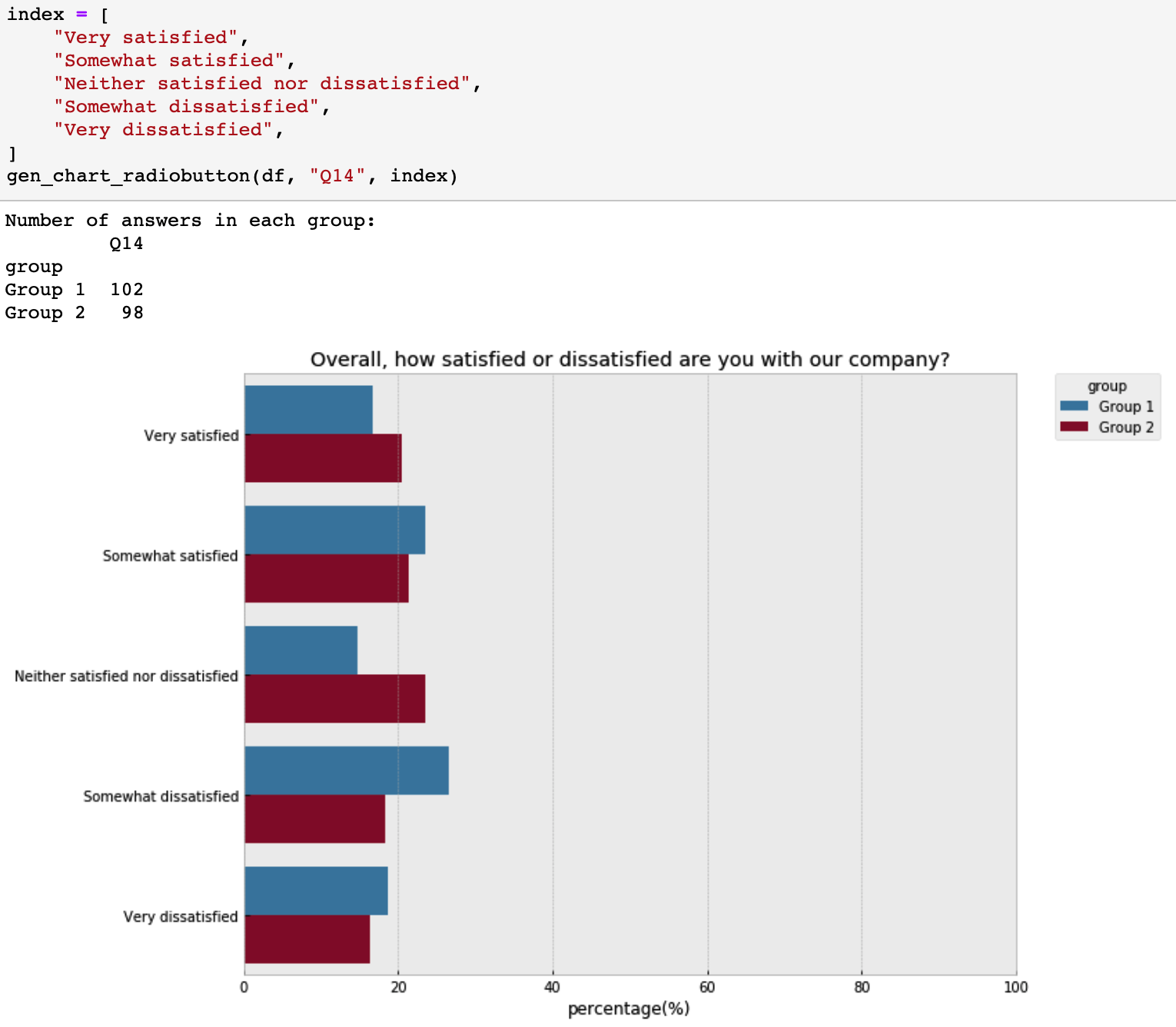### How to visualize the matrix table by group?

Example code:

# Generate summary data for each group
def gen_table(data, group_name, col_range, group_column_name='group'):
data_sub = data[data[group_column_name] == group_name].iloc[:, col_range].dropna(how="all")
for var in data_sub.columns:
data_sub[var] = data_sub[var].map(
{
"Strongly agree": 5,
"Agree": 4,
"Undecided": 3,
"Disagree": 2,
"Strongly disagree": 1,
}
)
table = data_sub.describe().loc[["mean", "std", "count"]].T

index = []

for var in table.index:
i = int(var[1:])
index.append(codebook.iloc[i, 1])

table["item"] = index

# table["item_n"] = range(len(index),0,-1)
table["item_n"] = range(0, len(index))
return table

# Generate a chart to compare the importance of missing features across two groups
def compare_importance(data, groups, col_range, group_column_name='group'):

group_name_to_describe_data = {}

#Create a dictionary to save the summary data for each group
for i, group_name in enumerate(groups):
group_name_to_describe_data[group_name] = "data_describe_%s" % i

for group_name in groups:
table = gen_table(data, group_name, col_range, group_column_name)
group_name_to_describe_data[group_name] = table

#Get the item list and index
items = table.item.tolist()
item_n = table.item_n.tolist()

# Visualize the mean value with the 95% confidence interval
# Change the figsize if you have more yticks
plt.figure(num=None, figsize=(12, 6), dpi=90, facecolor="w", edgecolor="k")
ax = plt.axes()

for i, group_name in enumerate(groups):
plt.errorbar(
group_name_to_describe_data[group_name]["mean"].astype(float),
group_name_to_describe_data[group_name]["item_n"] - 0.1 * i,
xerr=1.96
* (
group_name_to_describe_data[group_name]["std"].astype(float)
/ (group_name_to_describe_data[group_name]["count"] ** 0.5)
),
fmt="o",
elinewidth=0.5,
capsize=4,
marker="o",
ms=4,
label=group_name,
)

ax.set_xlim(1, 5)

ax.set_yticks(item_n)
ax.set_yticklabels(labels=items, fontdict={'fontsize':16})

plt.title("Compare the mean values across groups (scale 1-5)", fontsize=16)

return plt.show()


Input:

• data: Name of the dataframe
• groups: Group names of surveys that you are interested in comparing
• col_range: A list of columns for the matrix question
• group_column_name: The name of the column to identify group in the string format

Note: Here please customize the function of score_to_numeric above in order to convert the text labels in the raw data into meaningful numbers that you can interpret.

Output: